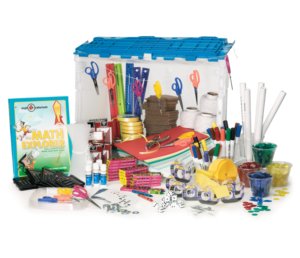# Math Explorer

## What is Math Explorer?

Math Explorer is a collection of creative, hands-on mathematics games and activities, designed to engage young people in standards-based mathematics learning and to support academic enrichment. The program invites middle school youth to develop, review, and practice important math skills while they engage in compelling projects, games, puzzles, art activities, and experiments. The program is designed to be easy to use by facilitators with any level of mathematical knowledge. It was created by the San Francisco Exploratorium, a nationally recognized leader in inquiry-based, hands-on education.

Math Explorer for grades 6–8 invites children to fly planes and launch rockets, play games, learn card tricks, and make cool stuff to take home. The program reinforces a variety of math skills, from problem-solving and graphing to working with fractions and ratios. Without the students realizing it, their math attitudes and aptitudes begin to change.Math Explorer addresses all 10 of the National Council of Teachers of Mathematics (NCTM) math-content and process areas.

##### Number and Operations
• Understanding the relationship between numbers
• Understanding the meanings of operation
• Computing fluently and make reasonable estimate
##### Algebra
• Representing and analyzing mathematical situations using algebraic symbols
• Using mathematical models to understand quantitative relationships
##### Geometry
• Analyzing geometric shapes
• Using coordinate geometry
• Applying transformations to analyze mathematical situations
• Using visualization and spatial reasoning to solve problems
##### Measurement
• Understanding the units and process of measurement
• Applying appropriate techniques and tools to determine measurements
##### Data Analysis and Probability
• Formulating questions and gathering data to answer them
• Using appropriate methods to analyze data
• Understanding and applying basic concepts of probability
##### Problem-solving
• Building new mathematical knowledge through problem solving
• Applying appropriate strategies to solve problems
##### Reasoning and Proof
• Making and investigating mathematical conjectures
##### Communication
• Organizing mathematical thinking through communication
• Communicating mathematical thinking clearly
• Evaluating the mathematical thinking of others
##### Connections
• Recognizing and applying mathematics in other contexts
##### Representation
• Using representations to organize and record mathematical ideas
• Using representations to model and interpret phenomena